# Describing Chemical Reactions Chapter 6 2 Page 224

• Slides: 13Describing Chemical Reactions Chapter 6. 2 Page 224Section 2: Describing Chemical Reactions What information does a chemical equation contain? How is matter conserved during a chemical reaction? What must a balanced chemical equation show? What are three types of chemical reactions?Chemical Formulas The chemical formula of a compound identifies the elements in the compound and the ratios in which their atoms are present. What do the following chemical formulas tell you?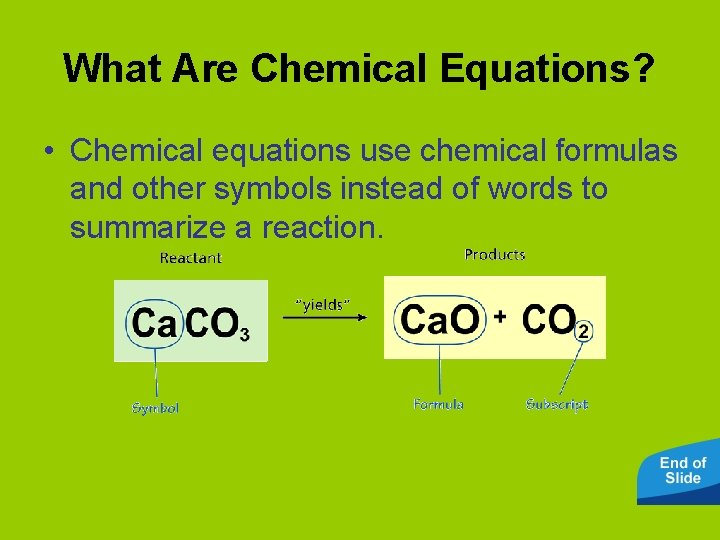What Are Chemical Equations? • Chemical equations use chemical formulas and other symbols instead of words to summarize a reaction.Conservation of Matter • The principle of conservation of matter states that in a chemical reaction, the total mass of the reactants must equal the total mass of the products.Conservation of Matter • In chemical reactions, the number of atoms stays the same. They are just rearranged to form different substances. 6 H 2 O + 6 CO 2 water + carbon dioxide C 6 H 12 O 6 + 6 O 2 glucose + oxygen PHOTOSYNTHESIS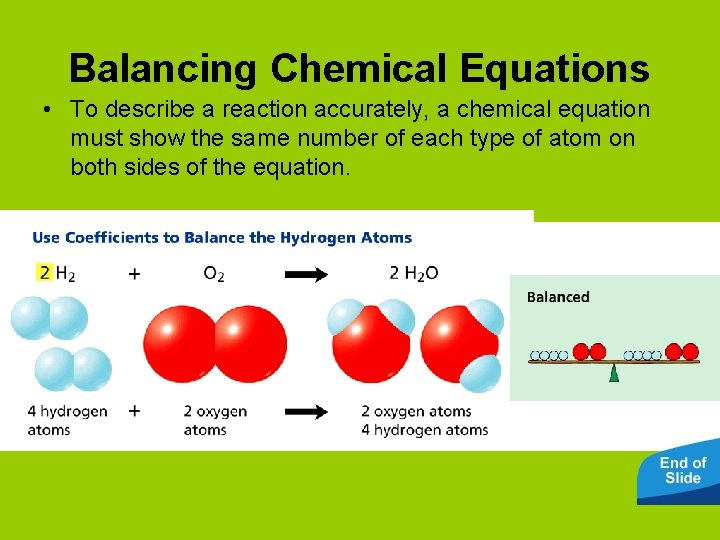Balancing Chemical Equations • To describe a reaction accurately, a chemical equation must show the same number of each type of atom on both sides of the equation.Balancing Equations Activity • Click the Active Art button to open a browser window and access Active Art about balancing equations.Balancing Chemical Equations • Magnesium (Mg) reacts with oxygen gas (O 2), forming magnesium oxide (Mg. O). To write a balanced equation for this reaction, first write the equation using the formulas of the reactants and products, then count the number of atoms of each element.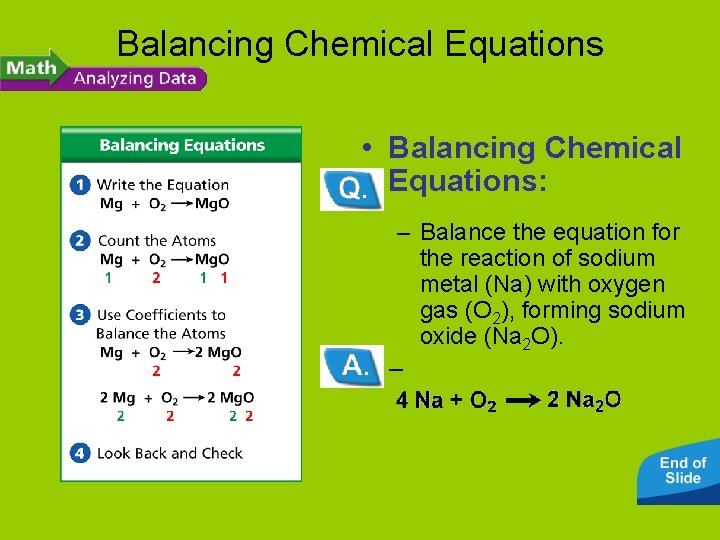Balancing Chemical Equations • Balancing Chemical Equations: – Balance the equation for the reaction of sodium metal (Na) with oxygen gas (O 2), forming sodium oxide (Na 2 O). –Balancing Chemical Equations • Balancing Chemical Equations: – Balance the equation for the reaction of tin (Sn) with chlorine gas (Cl 2), forming tin chloride (Sn. Cl 2). –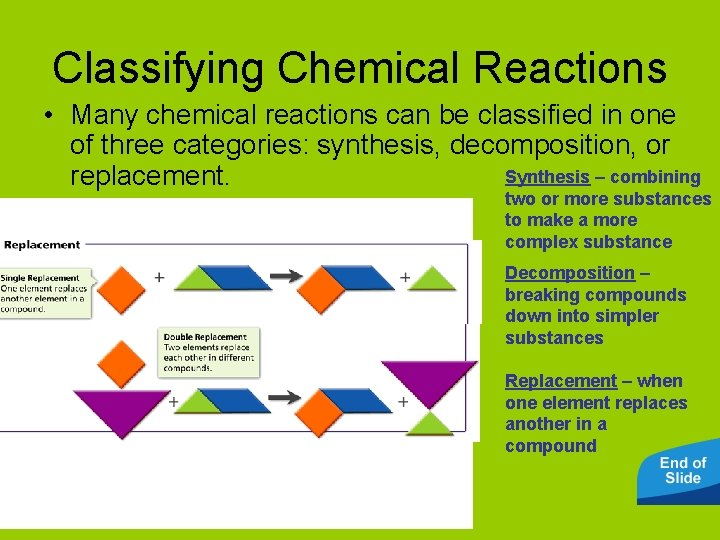Classifying Chemical Reactions • Many chemical reactions can be classified in one of three categories: synthesis, decomposition, or Synthesis – combining replacement. two or more substances to make a more complex substance Decomposition – breaking compounds down into simpler substances Replacement – when one element replaces another in a compound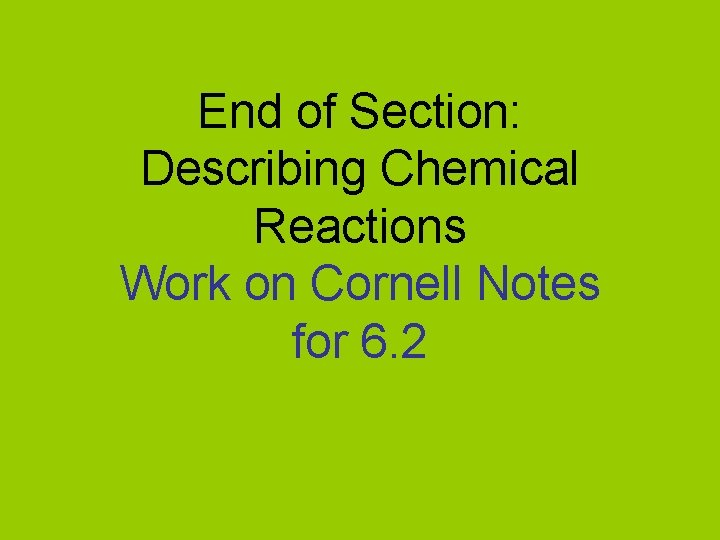End of Section: Describing Chemical Reactions Work on Cornell Notes for 6. 2